## Monday, January 31, 2011

### Jocelyn Leclaire Percent Video Post

A percent can be very useful in our life today. If we didn't have a percents in our life, our lives and business would be just horrible! It would be confusing, and well just plain out dufficult. One of the ways and the most easiest way to figure out a percent is to figure out the "silver bullet" as Mr.Harbeck calls it of a number.

Cylinder & Volume Post.Height = 10cm
Diameter = 20.3cm

r= d/2
r = 20.3 / 2
r = 10.5cm

v = π x r x r x h

v = 3.14 x 10.15 x 10.15

v = 323.49cm2
v = 323.49 x 10

v = 3234.9cm3Height = 10m
Diameter = 0.8 (inside) and 1 (outside)

Inside -
R = d/2
R = 0.8/2
R = 0.4

V = π x r x r x h
V = 3.14 x 0.4 x 0.4 x h
V = 0.5 x 10
V = 5m

Outside -
R = d/2
R = 1/2
R = 0.5

V = π x r x r x h
V = 3.14 x 0.5 x 0.5 x h
V = 0.78 x 10
V = 7.8m

### Final Percent Post Jocelyn Leclaire

In the chapter of Percents, Mr.Harbeck has taught me many things. He taught me that Percents are basically needed in the society of ours. He taught me about taxes, he taught me math and even some things about the real world! We learned about adding percents, estimate percents and like I said finding the tax on items and many other things in this unit.

Definition : A percent is a way to express a number into a fraction of a hundred.

My scribe post

Tutorial of Percents

### Cathlene's Surface Area Post

Question 2

(base x height ÷ 2 = area)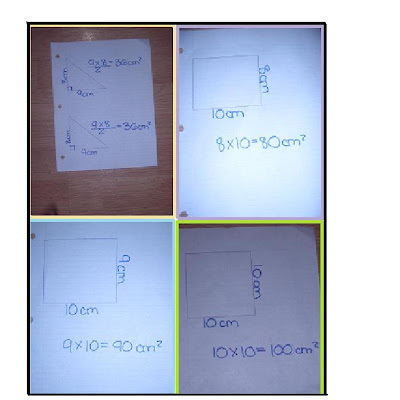TSA= 342 cm ²

Question 4

(lenght x width = area)TSA= 228 cm ²

## Sunday, January 30, 2011

### Winnipeg Harvest

On January 25th, 2011, 6 students from room 8-16 went to Winnipeg harvest for a couple hours during the afternoon. There, we met Leslie who taught us about Winnipeg Harvest and guided us around the buildings. Leslie taught us a lot about what Winnipeg Harvest does. She told us about the people they help as well as the types of food they hand out. Did you know that Winnipeg Harvest helps around 50000 people per month, half of which are children? How about how almost all the people who run Winnipeg Harvest are volunteers? Winnipeg Harvest is a food bank that distributes food that takes a LONG time to expire to people in need. Many of the items they receive are donated to them from people who want to help. Many stores also deliver dented cans and other products they are unable to sell to Winnipeg Harvest and there is even a bakery that gives freshly baked buns to them every morning! No wonder Manitoba was ranked the most generous province 12 times in a row! Although Winnipeg Harvest welcomes almost any type of food, they have a list of the top 10 foods that are most wanted. The types of food that they need the most are:

1. Canned fish and poultry
2. Canned fruits and vegetables (packed in own juice)
3. Canned stew, chili, brown beans
4. Peanut butter
5. Baby food
6. Whole grain/wheat pasta
7. Rice
8. Canned spaghetti sauce or tomatoes
9. Cereal
10. Canned soup

At Winnipeg Harvest, we packed rice into small bags. There were bins filled with rice, each with a different type. Two people were at each bin. One person at the bin was in charge of holding and tying the bags while the other scooped rice into the bags. The bags were then carried and put into boxes where they are later given to those who need it. I was one of the people in charge of holding and tying the bags. In the end, we bagged enough rice to help hundreds of families in Winnipeg.## Thursday, January 27, 2011

### Winnipeg Harvest :)

On Tuesday, January 25, 6 students, including myself went to Winnipeg Harvest and learned/ helped a lot that afternoon. When we first arrived there, Leslie talked to us about Winnipeg Harvest and what it's all about. Winnipeg Harvest is a food bank, that gives families food when they can't feed theirselves. She told us that there are thousands of people that come to Winnipeg Harvest and get food, because they can't afford to buy any. The Winnipeg Harvest team sends them a box of nutritient food that don't expire, like canned soup, canned beans and tomatoes, etc. Sometimes they give them bread and vegetables. They always give them an emergency bag that include rice or pasta, canned soup, canned vegetables and canned meat (I think). Winnipeg Harvest gets their food from a lot of different sources such as supermarkets that donate, and people who donate non perishable foods. Leslie told the group that a bakery always bakes an extra batch just for Winnipeg Harvest. The top 10 foods that they want to recieve are :
1. Canned soup
2. Peanut butter (light)
3. Canned fish
4. Rice
5. Cereal
6. Baby food
7. Canned fruits/ vegetables.
8. Rice
9. Whole grain pasta
10. Canned sauce.
When I volunteered at Winnipeg Harvest, the group and I helped pack rice into bags. I realized that every single piece of rice counts because these people aren't as lucky as we are to have food daily. We had packed about 25 bags of rice into 9-12 boxes. That meant we had helped up to 360 families.
Photos of us Winnipeg Harvest are one here .

## Wednesday, January 26, 2011

### Jayvee's Surface Area Scribe Post

2. A right rectangular prism has six faces. Why might you have to find the area of only three of the faces to be able to find the surface area? Use pictures and words to explain your thinking.

You only need to find the surface area of 3 faces because the top and bottom are the same, as well as the front and back, and the 2 sides.
.4. Find the surface area of this CD case.
Front: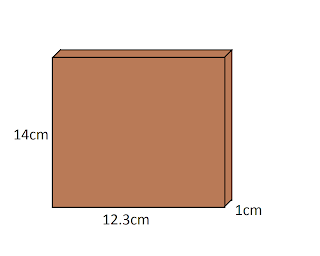14x12.3 = 172.2cm2
14x12.3 = 172.2cm2

Top :
12.3x1 = 12.3cm2
12.3x1 = 12.3cm2

Side :
14x1 = 14cm2
14x1 = 14cm2

TSA = 397cm2

6. Cheese is sometimes packaged in a triangular box. How much cardboard would you need to cover this piece of cheese if you do not include overlapping? Calculate your answer to the nearest tenth of a square centimetre.Front :
9.1x6.4 / 2 = 29.12cm2
9.1x6.4 / 2 = 29.12cm2

Top(Bottom) :
9.1x3 = 27.3cm2

Sides :
6.4x3 = 19.2cm2
6.4x3 = 19.2cm2

TSA = 123.94cm2

8. Paco builds a glass greenhouse.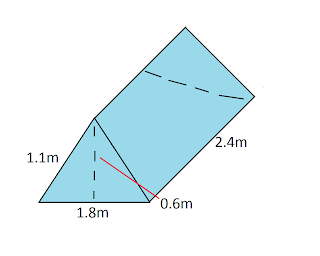A) How many glass faces does the greenhouse have?
The greenhouse have 5 faces.

B) How much glass does Paco need to buy?

Front :
1.8x1.1 / 2 = 0.99m2
1.8x1.1 / 2 = 0.99m2

Top (Bottom) :
2.4x1.8 = 4.32m2

Side :
2.4x1.1 = 2.64m2
2.4x1.1 = 2.64m2

TSA = 11.58m2

My Volume Question :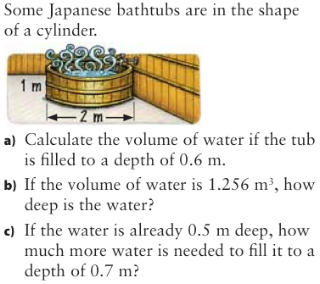Question from 7.3
A) d/2 = r
2/2 = r
1 = r
v = PiexRxRxH
v = 3.14x1x1x0.6
v = 3.14x0.6
v = 1.884cm^3
B) 1.256/1(h) = 1.256
1.256 / 1(r) / 1(r) / 3.14 = 0.4m
C) First, find the volume if the water's depth is 0.7m
3.14 x 1(r) x 1(r) x 0.7 = 2.198m^2
Now, find the volume if the water's depth is 0.5m
3.14 x 1(r) x 1(r) x 0.7 = 1.5 = 1.57m^2
After you get both of the answers, subtract 1.57 from 2.198 = 0.62m^m3

Question from 7.4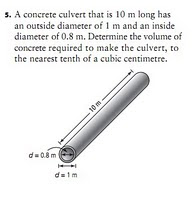First, find the volume of the bigger circle.
3.14 x 0.5 x 0.5 x 10 = 7.85m^2
Now, find the volume of the smaller circle.
3.14 x 0.4 x 0.4 x 10 = 5.024m^2
After you got both volumes, subtract 5.024 from 7.85
7.85 - 5.024 = 2.826m^3

### Winnipeg Harvest 2011

When all 6 of us kids went with Ms, Hay I was not expecting to do the rice by hand. Because when I went to Winnipeg Harvest in grade 5 we just put the food into boxes while it was moving. This experience was a good thing to do, I understood what Leslie was trying to get out. That not everyone has enough money to provide food and nutrition for their family. One of the fact I learned was that they could only give milk to children under 13 or 12, because they never had enough to provide for other children. Older sibling and parents would have to get powdered milk. When I was packing that rice into a small plastic baggy I was thinking about how this rice can and will make a difference on a child's life. Us 6 kids and Ms, Hay packed enough rice all by hand to be able to feed 360 families. This expiernce had a effect on me. And how I think about people in poverty, and not having the food, clothes and accesories provide for us. But thankfully we have it, and were extremely lucky.

Here is a photo of us at Winnipeg Harvest :http://www.flickr.com/photos/23046603@N00/5388616795/in/photostream/

## Thursday, January 20, 2011

### Final Percent Post (late)

Percent : it means out of 100 and it can also represent as a fraction or a decimal.

4.1 Representing Percents:

• To show a fractional percent between 0% and 1%, shade part of one square.
• One completely shaded grid represents 100%
• Means showing a percent as a picture in a hundred grids.

4.2 Fractions, Decimals, and Percents:

- Can be used to show numbers in different situations.

- Percents can be written as fractions and as decimals.

4.3 Percent of a number:

• · To calculate the percent of a number, write the percent as a decimal and then multiply by the number.
• · We can use mental math techniques such as halving, doubling, and dividing by 10 to find the percents of some numbers.

Example: 12 1/2 % of 50 = 0.275 x 50

= 13.75

Percent Video Questions:

4.4 Combining Percents:

· Percents can be put together by adding to solve problems.

Example: 5% + 7% = 12% ( We always use 12% as tax in our case)

· You can multiply the original number by a single percent greater than 100.

Example: 155% of 100 = 1.15 x 100

· You can add the combined percent amount to the original number.

Example: 15 % of 100 = 0.15 x 100 = 15

## Monday, January 17, 2011

### Final Percent Post

What is a percent?
A percent is a value out of 100, that can be expressed as a decimal, and a fraction. It is also another name for "hundredths".

- ex. 65% means 65 out of 100 or 65/100 or 0.65

Representing Percents (4.1)
To represent a percent, you can use hundred grids because they are out of 100.

- ex. 65%Fractions, Decimals, and Percents (4.2)
Percents can be represented as fractions and decimals. To convert a percent to a decimal, you must divide the percent by 100. To convert a decimal into a fraction, you write the decimal as the numerator. However, the denominator depends on the place value of the decimal.

eg.
decimal = 0.003 -> fraction = 0.003/1000 (because the 3 is in the thousandths place)
decimal = 5.98 -> fraction = 5.98/100 (because the 8 is in the hundredths place)

Now when the denominator is set, you must multiply the numerator until it becomes a whole number (or multiply it by the denominator).

eg.
0.003/1000 -> 0.003 x 1000 = 3/1000
5.98/100 -> 5.98 x 100 = 598/100

Lastly, you must simplify your fraction.

Percent of a Number (4.3)
To find a percent of a number, you can use mental techniques like halving, doubling, and dividing by ten.

When calculating the percent of a number, convert the percent to a decimal, and multiply it by the number.

ex. 12 1/2% of 50 -> 0.125 x 50 = 6.25
12 1/2% of 50 = 6.25

Combining Percents (4.4)
To solve certain problems, percents can be added together to form one percent.

ex.
GST + PST = taxes
GST = 5% PST = 7%
taxes = 5% + 7%
taxes= 12%

There are two ways to calculate the increase of a number. They are:

a) Adding the combined percent value to the original number.

eg. 12% of 100 -> 0.12 x 100 = 12
12 + 100 = 112

b) Using a singel percent greater than 100, and multiplying that to the original number.

eg. 112% of 100 -> 1.12 x 100 = 112

### Final Percent Post

4.1 Representing Percents : When representing percents , there's many ways you can show your percent . The easiest way is through a 100 grid . Mostly because a percent is out of 100 .

Example : 80/100 . To represent that you draw a 100 grid and shade in 80 Squares .

4.2 Fractions , Decimals & Percents : This is when you use various ways to convert Percents to fractions or decimals . There are many ways you could do this . One way you could find percent is by Multiplying or Dividing a number by 100 . To find the percent out of 100 .

Example : 30% of 100 . 30/100 = 0.3 0.3x100= 30

4.3 Percent of a number : Where you find a percent out of any number , It could be more than 100 or less that 1% .

Example : 3/4% = 0.75 . 150% of \$10 = \$15.00

4.4 Combining Percents : This is where you add to a Percent . As in , adding more to the additional percent . Having more than the regular percentage .

Example : Tax in Canada = 12% . If something cost \$10.00 .

10.00x1.12=\$11.20

Percent - is a number out of 100
- can also be represented as a decimal or a fraction

4.2 Representing Percents

You can represent a percent by shading squares on a hundred grid. A grid that is fully shaded means 100%. Use 2 or more hundred grids to show a percent larger than 100%. If you are shading less than 1%, shade a part of one square to represent it.

4.2 Fractions, Decimals, and Percents

Percent can also be written as a fraction and decimal. To change a percent into decimal, divide the percent by 100 to get the decimal. For changing into fraction, it is easier to use a decimal because it depends on how many digits there are after the decimal.

Example : 0.157 = 157/1000.

4.3 Percent of a Number

Doubling , halving, and dividing by ten are some strategies to find the percent of a number. Another way is converting the percent into a decimal then multiply it by the number.

Example : 12½ of 50 = 0.125
0.125 x 50 = 6.25

4.4 Combining Percents

Adding percents can solve problems, Example : 5% + 7% = 12%. To calculate the increase in a number, you can either:
-Add the percent amount to the original number.
Example : 12% of 100 = 0.12x100=12, 100 + 12 = 112
-Multiply the original number by a single percent greater than 100.
Example : 112% of 100 = 1.12 x 100

### Final Percent Post

The word percent means out of 100. Percents can be converted into decimals or fractions.
Ex. 65% - 65/100 - 0.65

Representing Percents 4.1

you can represent percents by using a hundred grid, since percents are out of 100.

Ex. this grid shows 13% since 13 squares are colored in.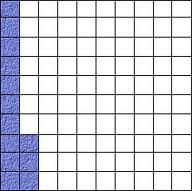Fractions, Decimals, and Percents 4.2

Percents can be converted into fractions, or decimals.
Ex. 50% - 1/2 - 0.5

Percent of a number 4.3

To calculate the percent of a number, write the percent as a decimal and then multiply by the number.
Ex. 12 1/2 % of 50 = 0.125 x 50
=6.25

Combining Percents 4.4

Combine percents by adding to solve the problems.
Ex. 5% + 7% =12%

MY PERCENT VIDEO

## Sunday, January 16, 2011

### Final Percent Post

Percent- means out of 100, it can also be written as a decimal or fraction.

Key points of chapter 4

Representing percents- you can represent percents by using a hundred grid, use one grid if the percent is under a hundred. Or 2 girds if over a hundred, to show a decimal you can shade part of a square in the grid.

Fractions, Percents, and Decimals- decimals can be written as a percent or fraction. Decimal to percent is multiplying by a hundred, and decimal to fraction is writing the decimal over 1 then multiplying the numerator and denominator by 10 for every number after decimal.

Percent of a number-you can find percents of a number by doubling,halving, or dividing by ten.

Combining Percents- percents can be combined by adding to solve problems (5% +7%=12%). To calculate the increase in a number, you can add the combined percent to the original number (12% of 100 = 0.12 x 100=12 100 + 12 = 112). You can also multiply the original number by a single percent greater than 100. (112% of 100 = 1.12 x 100 = 112).

### Karen's Final Percent Post

What is a Percent?
•number out of a hundred (50% means 50 out of a hundred, 50/100 or 0.50)

4.1 Representing Percents:
To represent a percent you can use 100 grids. One shaded grid that has all the squares shaded in represents 100%.
To show a percent that is larger then 100% you shade in more then on grid.
To represent a fractional percent between 0% and 1% you shade in a part of one square.
To represent a fractional percent greater than 1% shade squares from a hundred grid to show the whole number and part of on square from the grid to show the fraction.

4.2 Fractions, Decimals, Percents:
Fractions, decimals and percents can be used to represent numbers in various situations.
Percents can be written as fractions and decimals.
Example:
1/2% = 0.5% 150% = 150/100 42 3/4% = 42.75%
0.5 = 0.5/100 = .005 1.5 or 1 1/2 42.75% = 42.75/100 = 0.4275

4.3 Percent of a Number:
You can use some mental math stratagies such as doubling, halving, doubling or dividing by ten to find percents of some numbers.
To calculate the percent if a number write the percent as a decimal and then multiply by the number.
Example: 12 1/2% f 50 = 0.125 x 50 = 6.25 ( 12 1/2% = 12.5% = 0.125

4.4 Combining Percents:
Percents can be combined by adding to slove problems (eg: 5% + 7% = 12%).
To calculate the increase of a number:
-You can add the combined percent amount to the original number (eg: 12% of 100 = 0.12 x 100 = 12).
-You can multiply the original number by a single percent greater than 100 (eg: 112% of 100 = 1.12 x 100 = 112).
Percents of percents can be used to determine amounts that result from consecutive percent increases or decreases.

A website that further explains percent
A video that explains percent of a number
Another video that explains percent word problems

### Final Percent Post

What is a percent? A percent is a way to show a number out of 100. You can use the sign '%' to show percent. Examples: 50%, it is also equal to 50/100 as a fraction or 0.5 as a decimal.

In our recent unit on percent, we learned how to represent percents in different ways, convert them into decimals and fractions, how to calculate the percent of a number, and how to combine percents.

Representing Percents:
- To represent a percent, you can shade squares on a grid of 100 squares called a hundred grid. One completely shaded grid represents 100%.100%

- To represent a percent greater than 100%, shade more than one grid.-To represent a fractional percent between 0% and 1%, shade part of one square.- To represent a fractional percent greater than 1%, shade squares from a hundred grid to show the whole number and part of one square from the grid to show the fraction.
Fractions, Decimals, Percents:
- Fractions, decimals, and percents can be used to represent numbers in various situations.

- Percents can be written as fractions and as decimals.Percent of a Number:

- You can use mental math strategies such as halving, doubling, and dividing by ten to find the percents of some numbers.

- To calculate the percent of a number, write the percent as a decimal and then multiply by the number.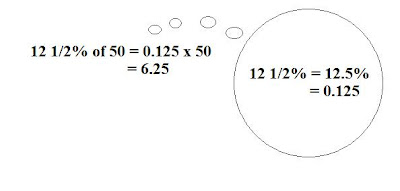Combining Percents:
- Percents can be combined by adding to solve problems. 5% + 7% = 12% .

- To calculate the increase in a number,

• You can add the combined percent to the original number. 12% of 100 = 0.12 x 100 = 12
• You can multiply the original number by a single percent greater than 100. 112% of 100 = 1.12 x 100 = 112

- Percents of percents can be used to determine amounts that result from consecutive percent increases or decreases.

Here is my video about percents.

Here is a link to my percent scribe post:

http://spmath81610.blogspot.com/search/label/Olivia816

http://www.math.com/school/subject1/lessons/S1U1L7GL.html

### Final Percent Post

Definition of percent:
any number out of 100,that can be represented as a fraction and a decimal.

Key Points of Chapter 4

4.1

When showing a percent greater than 100%, use more than 1 grid.
When showing a percent less than 1%, but more than 0%, use only one grid.

4.2

Percents can be shown as a decimal and fraction.
50% = 1/2 and 0.5

4.3
To calculate a percent of a number,write it as a decimal and multiply it.
15 1/2% of 50= 0.125x50
= 6.25

4.4
To combine a percent, put them,or add them together.
ex.1% + 2% = 3%
To found out the increase in a number, add the combined percent to the original number.
5% + 7% = 12%

### Jayvee's Final Percent Post

Percent - is a number out of 100
- can also be represented as a decimal or a fraction

Representing Percents

You can represent a percent by shading squares on a hundred grid. A grid that is fully shaded means 100%. Use 2 or more hundred grids to show a percent larger than 100%. If you are shading less than 1%, shade a part of one square to represent it.

Fractions, Decimals, and Percents

Percent can also be written as a fraction and decimal. To change a percent into decimal, divide the percent by 100 to get the decimal. For changing into fraction, it is easier to use a decimal because it depends on how many digits there are after the decimal.

Example : 0.157 = 157/1000.

Percent of a Number

Halving , doubling, and dividing by ten are some strategies to find the percent of a number. Another way is converting the percent into a decimal then multiply it by the number.

Example : 12½ of 50 = 0.125
0.125 x 50 = 6.25

Combining Percents

Adding percents can solve problems, Example : 5% + 7% = 12%. To calculate the increase in a number, you can either:
-Add the percent amount to the original number.
Example : 12% of 100 = 0.12x100=12, 100 + 12 = 112
-Multiply the original number by a single percent greater than 100.
Example : 112% of 100 = 1.12 x 100

### Final Percent Post

What is a percent?

-Means out of 100

-Another name for hundreths

-Ex. 76% means 76 out of 100

4.1 Representing Percents

-You can represent a percent by shading in a hundred grid

-You can also use more than one grid to represent a percent larger than 100

-If representing a fractional percent, you can shade in a part of one square

Ex.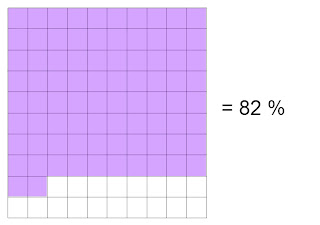4.2 Fractions, Decimals, and Percents

-Fractions, decimals, and percents can be used to represent numbers in various situations

-Percents can also be written as fractions and decimals

Ex. 50% = 0.5 = ½

4.3 Percent of a Number

-To find percents of some numbers, you can use mental math strategies such as halving, doubling and dividing by ten

-Write a percent as a decimal and multiply it by the number to find the percent of a number

Ex. 12 ½ % of 50 = 0.125 x 50 = 6.25

4.4 Combining Percents

-You can add some percents in order to solve a problem

-To find the increase of a number,

-You can add the combined percent amount to the original amount

Ex. 19% of 100 = 0.19 x 100 = 19

100 + 19 = 119

- You can multiply the original number by a single percent greater than 100

Ex. 119% of 100 = 1.19 x 100 = 119

This is my percent video:

### Paulo's Percent Review Video

A percent means out of 100.
Ex: 50% means 50/100, 50 out of 100, or 0.50

4.1Representing Percent

Representing percents means showing a percent as a picture in a hundred grid.
Ex. 50% on a hundred, 50 squares are shaded in a hundred grid so it would be 50%.

4.2 Fraction,Decimal,and Percents

Fractions,decimals, and percents can be used to represent numbers in various situations
-Percents can be written as fractions and as decimals.
Ex. 1/2%=0.50%, 0.5/1000=o.005, 150%=150/100=1.5 or 1 1/2

4.3 Percent of a number

-You can use mental math strategies such as halving,doubling, and dividing by ten to find the percents of some numbers.
-To calculate the percent of a number,write the percent as a decimal and then multiply by the number.
Ex. 12 1/2% of 50=0.125 x 50=6.25 ( 12 1/2 %=12.5%=0.125)

4.4 Combining Percent

-Percent can be combined by adding to solve problems. 5% + 7%=12%
-To calculate the increase in a number.
Ex. 12% of 100= 0.12 x 100=12,100 + 12=112
-You can multiply the original number by a single percent greater than 100
Ex. 112% of 100=112 x 100 = 112

Percent of percents can be used to determine amounts that result from consecutive increases or decreases.

This is my percent preview video:

## Saturday, January 15, 2011

### Lizelle's Percent Scribe.

For this scribe, I chose question #3, #8, and #12.

For #3 I got:
a) 6000
b) 6.15
c) 0.04

For #8 I got:
\$21.42

For #12 I got
1110 kilometres on a full tank.

Cylinder & Volume Post.Height = 10cm
Diameter = 20.3cm

r= d/2
r = 20.3 / 2
r = 10.5cm

v = π x r x r x h

v = 3.14 x 10.15 x 10.15

v = 323.49cm2
v = 323.49 x 10

v = 3234.9cm3Height = 10m
Diameter = 0.8 (inside) and 1 (outside)

Inside -
R = d/2
R = 0.8/2
R = 0.4

V = π x r x r x h
V = 3.14 x 0.4 x 0.4 x h
V = 0.5 x 10
V = 5m

Outside -
R = d/2
R = 1/2
R = 0.5

V = π x r x r x h
V = 3.14 x 0.5 x 0.5 x h
V = 0.78 x 10
V = 7.8m

### Final Percent Post

In this chapter/unit I have learned many things about percent. Such as that you can add percents together, you can divide percents, that you can estimate percents, finding taxes on items and many more things. Percents can be really handy in life, especially finding the taxes on an item.

Definition : A percent is a way to express a number into a fraction of a hundred.

My scribe post:

A thread that many people explain percents.

### Lizelle's Percent Review Video

A percent is a way to express something in a fraction of a percent. You can find the percentage of something very easily. The easiest way to find a percent is to find the "silver bullet." Percent's can be very useful in our life, it's also very helpful if we need to find out the fraction of something or a number.

## Friday, January 14, 2011

### Final Percent Post

Percent
a percent means out of 100
a percent can be represented as a fraction, decimal and in a hundred grid

Key Points of Chapter 4
4.1

When representing a percent greater than 100%, use more than 1 grid.
When representing a percent less than 1%, but more than zero, shade a part of one square.
4.2
Percents can be represented as a fraction, and a decimal.
1/2 = 50% = 0.50
4.3
To calculate a percent of a number, write the percent as a decimal then multiply the number
12 1/2% of 50= 0.125x50
= 6.25
4.4
To combine a percent, add them together.
To found out the increase in a number, add the combined percent to the original number.
5% + 7%(taxes) 12%

My percent video:

My Percent Scribe

### Final Percent Post

Percent means out of a hundred. It's another name for hundredths. They can be represented as a fraction or a decimal.
This chapter, we did percents. We have learned a lot from, showing percents on a hundred grid(s), converting them into decimals and fractions, combining percents and a percent of a number.

We first learned about representing percents. You can have percents from less than 0 to more than 100 on a grid . The percents involve fractions as well.
An example of a number on a hundred grid, containing more than 100 is:An example of a number on a hundred grid, containing less than 100 is:The next thing we learned about fractions were converting them into fractions and decimals.
Example: convert the following into a decimal and percents .
a) 3/40 = 0.075
the numerator divided by the denominator and you get the decimal .
b) 0.075 x 100 = 7.5

4.3 was finding a percent of a number. I like to use the ratio grid to find the percent .
example: whatever you do one side, you do to the other side.The last thing we learned about percents were combining them . I thought this was really easy.

### Final Percent Post

What is a percent? A percent is out of a hundred. It is also another way of saying hundredths.

We recently learned about percents and did many things with them. We learned how to represent them in different ways, change them into fractions, decimals, and percents, how to find a percent of a number and how to combine percents.

Representing Percents:
You can use hundred grids to represent percents. You can also use more than one grid and shade part of a square.
Example: If you had to represent 180%, you would shade in one whole one hundred grid and 80 squares of another.

Fractions, Decimals, Percents:
You can use fractions and decimals to represent percents.
Example: 1/2 = 0.5 or 50%.

Percent of a Number:
To find the percent of a number, you can use some simple mental math strategies such as halving, doubling and dividing. You can also take the number and change it to a decimal then multiply that by the other number given.
Example: If you were finding 160% of \$53.27...
1.60 x 53.27 = \$85. 23

Combining Percents:
Percents can be combined by adding to help you solve problems. You can also use other strategies such as multiplying.
Example:
Ravi purchased 3 DVDs for \$19.99 each.
Find the total cost for the DVDs including
5% GST and 6% PST.

\$19.99 x 3 = \$59.97
\$59.97 x 1.11 = \$66.5667
= \$ 66.57

Here is my math video answering the four questions Mr. Harbeck gave us.

This is my percent post.
Here is a video that I hope will help you further understand percents.

## Thursday, January 13, 2011

### Tyler's Percent Scribe Post

4.Use mental math to do the following.

a)20% of 60= 12

b)250% of 400= 1000

c)10 1/2 % of 100=10.5

8.The original price of a jacket was \$84.00. A store manager marked the price down by 25 1/2 %. By how much was it reduced?

84 x 0.255 = \$21.42

11. Josephine haad an average of 40% after her first game, the second game she had ten shots and finished with an average of 50%. How many baskes did she make out of ten.
She made 8 shots out of ten.

### Final Percent Post

What is a Percent?
Percent is a number out of 100.
For example 50% is 50 out of 100.

Percent can be turned into a decimal and to a fraction.
For example 50% in decimal will be 0.5 and in fraction it's going to be 1/2.

If your turning a percent to a decimal, divide the percent by 100 and you'll get your answer

If your turning a percent to a fraction first find the decimal of the percent, second count how many digits you have after the decimal point then put zero's every digit you have after the decimal.
E.G 45%=0.45=45/100

Percents can be represent also by shading square from a hundred grid. To represent 100% in to a hundred grid color all of the squares. To represent a percent that is less than 1% shade part of one square then draw another square and divide that square whatever is the denominator given to you.

Percent have a strategies like halving, doubling, and dividing by ten if you are to ask use mental math.

Here is my video for the percent questions